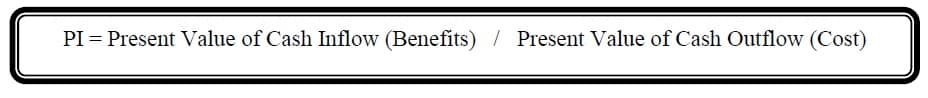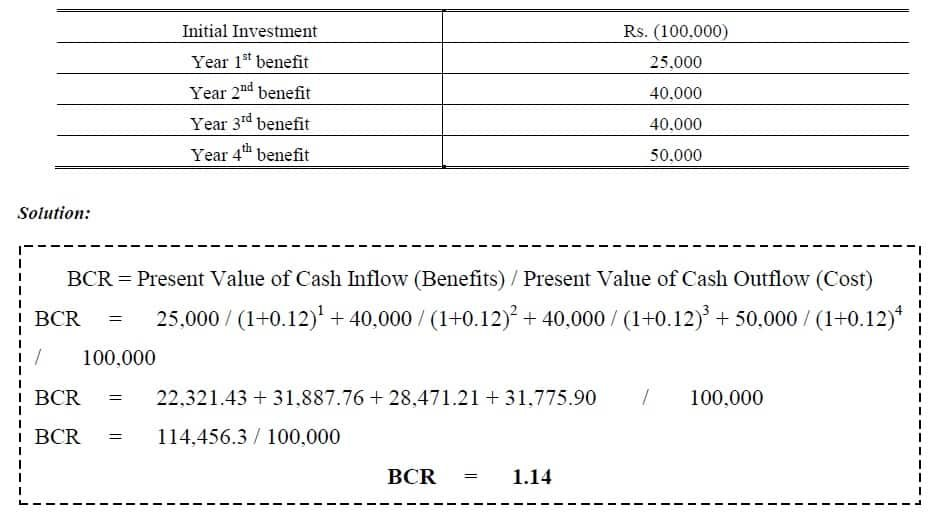Select Page

# Profitability Index (PI)

Profitability Index is also called Benefit Cost Ratio (BCR), is the sum of all present values of cash inflows that are expected to occur over the life of project divided by present values of all cash outflows. So we can say that Benefit Cost Ratio is ratio between Cash Inflow and Cash Outflow:Accepted Criteria when BCR                                           Rule is

> 1                                                                          Accepted

= 1                                                                          Indifference

< 1                                                                            Rejected

### Example 1:

Find the Profitability Index if cost of capital is 12 %?(Acceptance Criterion: The higher the BCR the better the project)